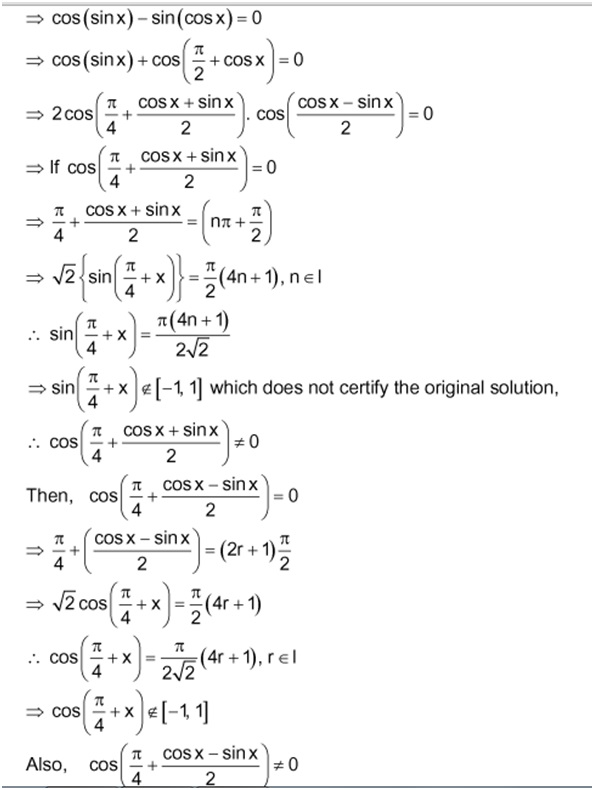# Needed a short method

Prove that $sin(cosx)=cos(sinx)$ has no real solution.

The method i came across was thisLHS is not equal to RHS.

If u have got any other method do post it.Note by Tanishq Varshney
6 years, 1 month ago

This discussion board is a place to discuss our Daily Challenges and the math and science related to those challenges. Explanations are more than just a solution — they should explain the steps and thinking strategies that you used to obtain the solution. Comments should further the discussion of math and science.

When posting on Brilliant:

• Use the emojis to react to an explanation, whether you're congratulating a job well done , or just really confused .
• Ask specific questions about the challenge or the steps in somebody's explanation. Well-posed questions can add a lot to the discussion, but posting "I don't understand!" doesn't help anyone.
• Try to contribute something new to the discussion, whether it is an extension, generalization or other idea related to the challenge.
• Stay on topic — we're all here to learn more about math and science, not to hear about your favorite get-rich-quick scheme or current world events.

MarkdownAppears as
*italics* or _italics_ italics
**bold** or __bold__ bold
- bulleted- list
• bulleted
• list
1. numbered2. list
1. numbered
2. list
Note: you must add a full line of space before and after lists for them to show up correctly
paragraph 1paragraph 2

paragraph 1

paragraph 2

[example link](https://brilliant.org)example link
> This is a quote
This is a quote
    # I indented these lines
# 4 spaces, and now they show
# up as a code block.

print "hello world"
# I indented these lines
# 4 spaces, and now they show
# up as a code block.

print "hello world"
MathAppears as
Remember to wrap math in $$ ... $$ or $ ... $ to ensure proper formatting.
2 \times 3 $2 \times 3$
2^{34} $2^{34}$
a_{i-1} $a_{i-1}$
\frac{2}{3} $\frac{2}{3}$
\sqrt{2} $\sqrt{2}$
\sum_{i=1}^3 $\sum_{i=1}^3$
\sin \theta $\sin \theta$
\boxed{123} $\boxed{123}$

## Comments

Sort by:

Top Newest

Look at the function $f(x) = \cos(\sin(x)) - \sin(\cos(x)).$ This function is even and periodic with period $2\pi.$ so we just need to focus on the interval $[0, \pi].$

Now $f(0) = 1 \gt 0.$ Also, for $x \in [\frac{\pi}{2}, \pi]$ we have $\cos(\sin(x)) \gt 0$ and $\sin(\cos(x)) \le 0,$ so $f(x) \gt 0$ on this interval.

So we now just need to focus on the interval $(0, \frac{\pi}{2}).$ On this interval we have that both $\cos(x)$ and $\sin(x)$ lie between $0$ and $1,$ for which $\sin(\cos(x)) \lt \cos(x) \lt \cos(\sin(x)),$, i.e., $f(x) \gt 0.$ Thus $f(x) \gt 0$ for all $x$.

(To expand on this last step, note that for $\theta$ in $(0, \frac{\pi}{2})$ we have $0 \lt \sin(\theta) \lt \theta,$ and so $\cos(\sin(\theta)) \gt \cos(\theta).$ )

- 6 years, 1 month ago

Log in to reply

Yes! I recall when I first saw bounding those values by $\cos x$, which seemed really ingenious to me.

Staff - 6 years, 1 month ago

Log in to reply

The easiest way for me is to plot the graph for the full range of $x \in [0,2\pi)$. The graph is as follows. We note that the $\sin{(\cos{x})} \ne \cos{(\sin{x})}$ always.- 6 years, 1 month ago

Log in to reply

Well, part of the question would then be "How do you know that's how the graphs should be drawn (Other than relying on a calculator / graphing capabilities)?"

Staff - 6 years, 1 month ago

Log in to reply

Do you mean how to do graphs with Excel spreadsheet? If so, maybe I should write a wiki on it. There are others requested for it. I just don't like to do it.

- 6 years, 1 month ago

Log in to reply

It is easy to see that $cos(cos(x))-sin(sin(x))=0$ has no solutions. Just replace $x$ with $\frac{\pi} {2}-x$

- 6 years, 1 month ago

Log in to reply

- 6 years, 1 month ago

Log in to reply

It's convincing that $cos(cos(x)) \ne sin(sin(x))$ but how can you prove that statement?

- 6 years, 1 month ago

Log in to reply

Sketch the rough graph of both the functions

- 6 years, 1 month ago

Log in to reply

×

Problem Loading...

Note Loading...

Set Loading...